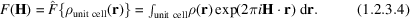International
Tables for
Crystallography
Volume B
Reciprocal space
Edited by U. Shmueli

International Tables for Crystallography (2010). Vol. B, ch. 1.2, p. 10   | 1 | 2 |

Section 1.2.3. Scattering by a crystal: definition of a structure factor

P. Coppensa*

aDepartment of Chemistry, Natural Sciences & Mathematics Complex, State University of New York at Buffalo, Buffalo, New York 14260–3000, USA
Correspondence e-mail: coppens@buffalo.edu

1.2.3. Scattering by a crystal: definition of a structure factor

| top | pdf |

In a crystal of infinite size,is a three-dimensional periodic function, as expressed by the convolution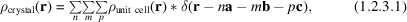where n, m and p are integers, and δ is the Dirac delta function.

Thus, according to the Fourier convolution theorem,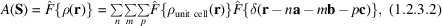which gives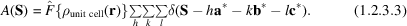Expression (1.2.3.3)is valid for a crystal with a very large number of unit cells, in which particle-size broadening is negligible. Furthermore, it does not account for multiple scattering of the beam within the crystal. Because of the appearance of the delta function, (1.2.3.3)implies that S = H with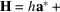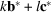.

The first factor in (1.2.3.3), the scattering amplitude of one unit cell, is defined as the structure factor F: